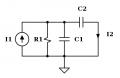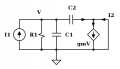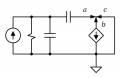# VCCS in parallel with a short circuit

#### thumb2

Joined Oct 4, 2015
122
It seems I have a problem with circuits containing dependent sources also because in my circuit course I did not deal with them so sometimes I have doubts I have to solve by my self.

This is a 2nd part of this thread.
The circuit is the samebut this time I tried to determine

$$h_{21} = \left . \frac{I_2}{I_1} \right |_{V_2 = 0} = \frac{g_m - sC_2}{G_1 + s(C_1 + C_2)}$$

and my doubt is related with the VCCS in parallel with a short-circuit.

It seems it's turned off in calculating $$h_{11}$$.

At first glance, I would say that a VCCS in parallel with a short-circuit has no effect on the rest of the circuit (same as a resistor in parallel with short-circuit) so the following circuitdisagrees with the book's solution, so while working on this circuit:applying the current divider the result agrees with the following reasoning:

\begin{align} I_2 = I_1\left(\frac{\frac{1}{sC_1} \parallel R_1 }{\left(\frac{1}{sC_1} \parallel R_1 \right ) + \frac{1}{sC_2}} \right ) - g_mV_1 = I_1\left(\frac{\frac{1}{sC_1} \parallel R_1 }{\left(\frac{1}{sC_1} \parallel R_1 \right ) + \frac{1}{sC_2}} \right ) - g_m I_1\left( R_1 \parallel \frac{1}{sC_1} \parallel \frac{1}{sC_2} \right ) \end{align}

So the question is: if the VCCS has been turned of off calculating $$h_{11}$$, why shouldn't be turned off calculating $$h_{21}$$ ? This is a non-sense because these are two different behaviours for the same situation (VCCS in parallel with a short-circuit) , and of course I am wrong and my reasoning is not correct.

Could you help me to understand ?
thumb2

#### thumb2

Joined Oct 4, 2015
122
Oh well ... in $$h_{11}$$ case, $$g_{m}V$$ is equal to the current flowing in C2 so can be neglected.

I understand that the VCCS should not be turned off when short-circuited.
Otherwise I am quiet confused.

#### MrAl

Joined Jun 17, 2014
9,622
Hi,

I have to applaud you on the very well formed presentation of your question. You have drawn out all the necessary circuits to understand what you are doing and what you are asking and maybe what seems like it is not working right.

If you analyze the circuit and calculate I2 and I1 and then divide I2/I1 you will get:
I2/I1=(gm-s*C2)/(G1+s*C1+s*C2)

If you analyze for voltage instead of current for example, you can use R1||C1||C2 as the input impedance Zin so then I1=Vin/Zin because the output is a short for voltage.
Because the short is in parallel to R2, you can then keep R2 in the circuit for the calculation of I2, then later take the limit as R2 goes to zero, and that would be the case when the output is shorted because 0 ohms in parallel to any finite resistance is zero.

So that gives you both I1 and I2 and then you can easily calculate I2/I1.

So here the only things you have to know is how to analyze the circuit for an input voltage and no output load, and how to find the input and output current from that analysis when the output is shorted.
If you feel uncomfortable with using R2 in a dual role, then just add another resistor R3 to the output as the load and let that go to zero in the calculation instead of R2. You probably then have to watch out for a sign change though.

BTW, doing the above then you can also easily calculate V1/I1:
V1/I1=1/(G1+s*C2+s*C1)

What else you could do is use three current sources, adding current sources I1 and I2, then solve for those two current sources and that gives you I1 and I2.

The short answer is since all these techniques work, then you should not try to think of gm as being 'turned off' at any time. Rather analyze the circuit the way you would any other circuit and just calculate I1 and I2 somehow and then you can divide. Of course at some point you have to set the output V2 to zero, but that's only AFTER you solve for the required quantities.
A 'short' in circuit analysis is sometimes a little tricky. Sometimes it is a short at one point in the analysis and not a short at another point in the analysis. Usually the difference is when analyzing for a voltage vs for a current.

Last edited:
•thumb2

#### thumb2

Joined Oct 4, 2015
122
MrAl said:
I have to applaud you on the very well formed presentation of your question ...
Thank you.

MrAl said:
If you analyze the circuit and calculate I2 and I1 and then divide I2/I1 you will get:
I2/I1=(gm-s*C2)/(G1+s*C1+s*C2)
Yes, however the correct equation I wrote in post #1 should be:

\begin{align} -I_2 = I_1\left(\frac{\frac{1}{sC_1} \parallel R_1 }{\left(\frac{1}{sC_1} \parallel R_1 \right ) + \frac{1}{sC_2}} \right ) - g_m I_1\left( R_1 \parallel \frac{1}{sC_1} \parallel \frac{1}{sC_2} \right ) = I_1\left(\frac{sR_1C_2}{sR_1C_1 + sR_1C_2 + 1} \right ) - g_m\left(\frac{I_1R_1}{sR_1C_1 + sR_1C_2 + 1} \right )\\ \end{align}

$$\frac{I_2}{I_1} = \frac{g_mR_1 - sR_1C_2}{sR_1C_1 + sR_1C_2 + 1} = \frac{g_m - sC_2}{sC_1 + sC_2 + G_1}$$

where of course $$G_1 = 1/R_1$$. This agrees with the result of the book.
I think the resolution (not only the final result) it's correct since given this circut example (where I called the currents at the node a, b & c)whe have for simple KCL that

$$-a - b = c$$

and since b is negative because b = -gmV, it brings to the correct result.

Last edited:

#### MrAl

Joined Jun 17, 2014
9,622
Hi,

Yes a mistake in the math is always a possibility. It helps to go back to basics when you cant figure out why something isnt working, and so some standard method like nodal analysis is always a good idea. If you get two different results, something is not right in one of them•thumb2